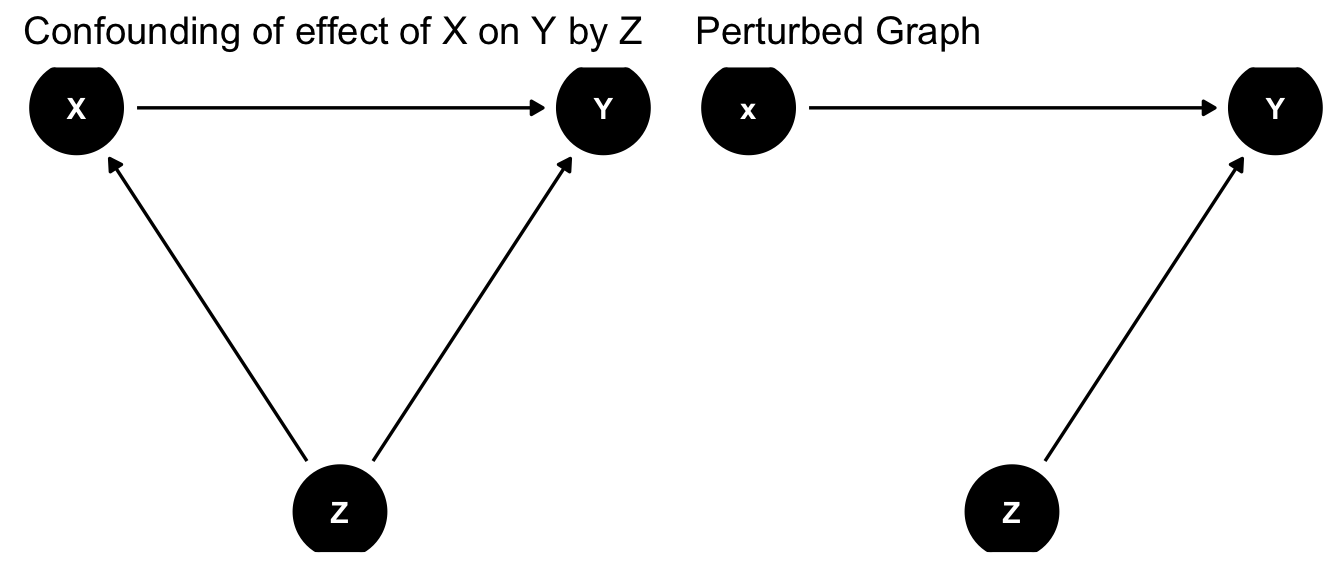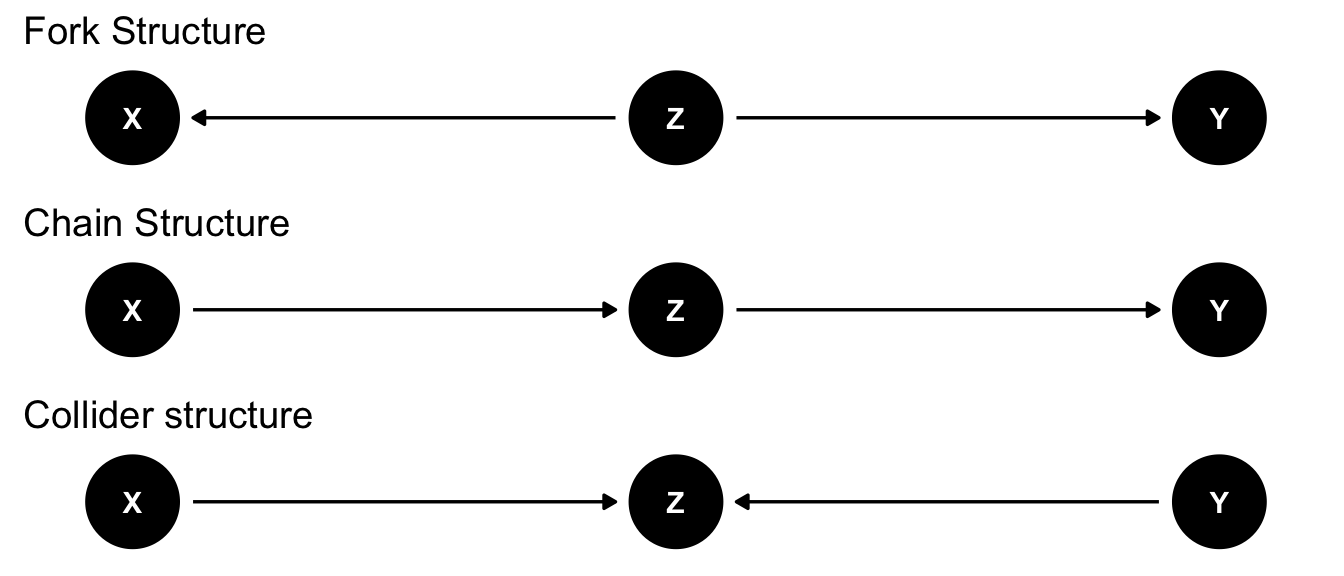#Libraries for causal graphs
library(dagitty) #Identification
library(ggdag) #plotting
library(pcalg) #search
library(gridExtra) #Graph Display

## Plans

• Structural equations models provide a complete framework for answering questions about observed, interventional, or counterfactual quantities

• Given a model definition, a characterization of distributions of variables as defined by the “solution” of that model, and characterization of causation as that distribution in a modified model, you can derive formulas for distribution of any observed or counterfactual quantity

• Given a quantity defined in terms of features of the causal model, identification corresponds to finding a formula in terms of observed quantities which is identical to the causal model quantity, or certifying that no such formula exists

• E.g., causal quantity is ATE $$E[Y^{X=x_1}-Y^{X=x_0}]$$, identification formula is $$\int (E[Y|X=x_1,Z=z]-E[Y|X=x_0,Z=z])dP(z)$$
• Actually deriving the identification result may be challenging: search space can be large or infinite

• For restricted model classes, relationships can be described by set of rules, via which search can be automated
• This has been done comprehensively for acyclic Structural Causal Model with independent errors
• Representable by a causal Directed Acyclic Graph or DAG
• Today: Brief summary of known identification results for DAGs

• Model assumptions let you convert qualitative reasoning about which variables are related to which others and how into estimation formulas and observables implications
• Will only reference extensions to models with weaker or stronger assumptions
• Most immediate payoff is a framework for reasoning about conditional ignorability $$Y^x\perp X |Z$$ called backdoor criterion

• Highlights reasons why a regression may or may not recover a causal effect
• Secondarily, generate alternative estimation formulas and model tests

• Since methods automated, focus will be on interpreting assumptions and results, less on derivations

## Structural Causal Models and DAGs, review

• Endogenous variable $$Y_1,Y_2,\ldots,Y_p$$ described by Structural Equation Model $Y_1=f_1(Y_2,\ldots,Y_p,U_1)$ $Y_2=f_2(Y_1,Y_3,\ldots,Y_p,U_2)$ $\vdots$ $Y_p=f_p(Y_1,Y_2,\ldots,Y_{p-1},U_p)$
• Exogenous $$(U_1,\ldots,U_p)\sim\Pi_{j=1}^{p}F_j(u_j)$$ mutually independent
• Variables $$Y_1,\ldots,Y_j$$ encoded as nodes $$V$$ in graph $$G=(V,E)$$
• Presence of $$Y_j$$ in $$f_i$$ indicates $$Y_j$$ directly affects $$Y_i$$
• Encoded as edge $$Y_j\to Y_i$$ in $$E\in V\times V$$
• “Acyclic”: no directed path (sequence of connected edges with common orientation) from a vertex to itself
• “Nonparametric”: graph topology encodes only presence or absence of connection
• “Solve”: Define $$(Y_1,\ldots,Y_p)$$ as unique values that solve system given $$U$$’s
• $$do(Y_j=x)$$”: Replace $$f_j$$ by $$x$$, solve. New values are $$(Y_1^{Y_j=x},\ldots, Y_{j-1}^{Y_j=x},x,Y_{j+1}^{Y_j=x},\ldots,Y_{p}^{Y_j=x})$$

## Causal Markov Condition

• Solving an acyclic structural model gives joint distribution of endogenous variables
• What properties does the joint distribution have?
• Causal Markov property: A variable $$Y_j$$ is independent of any variable that is not a descendant, conditional on its parents
• $$Y_k$$ is a parent of $$Y_j$$ if there is a directed edge from $$Y_k$$ to $$Y_j$$. $$pa(Y_j)$$ is the set of parents of $$Y_j$$
• $$Y_k$$ is a descendant of $$Y_j$$ if there is a path along directed edges from $$Y_j$$ to $$Y_k$$
• Property completely defines implications of causal graph
• Absence of an edge means conditional independence
• Implies that joint distribution factorizes according to topological order of graph
• $$P(Y_1,\ldots,Y_p)=\Pi_jP(Y_j|pa(Y_j))$$
• When intervening causally, $$f_j$$ is deleted but rest of structure, including distributions, remains the same
• Joint distribution given $$do(Y_j=x)$$ is $$P(Y_1,\ldots,Y_p|do(Y_j=x))=(\delta_{Y_j=x})\Pi_{i\neq j}P(Y_i|pa(Y_i))$$
• $$\delta_{Y_j=x}$$ is point mass at $$x$$, every other part is unchanged
• Ratio of $$P(Y_1,\ldots,Y_p|do(Y_j=x))/P(Y_1,\ldots,Y_p)=\frac{\delta_{Y_j=x}}{P(Y_j|pa(Y_j))}$$ immediately recovers Inverse Probability Weighting formula
• IPW using parents is valid estimator for any causal effect

## Illustration: Adjustment Formula from Causal Markov

structuregraphs<-list()

confoundgraph<-dagify(Y~X+Z,X~Z) #create graph
#Set position of nodes
coords<-list(x=c(X = 0, Z = 1, Y = 2),y=c(X = 0, Z = -0.1, Y = 0))
coords_df<-coords2df(coords)
coordinates(confoundgraph)<-coords2list(coords_df)
structuregraphs[]<-ggdag(confoundgraph)+theme_dag_blank()+labs(title="Confounding of effect of X on Y by Z") #Plot causal graph
perturbedgraph<-dagify(Y~x+Z) #create graph
#Set position of nodes
coords<-list(x=c(x = 0, Z = 1, Y = 2),y=c(x = 0, Z = -0.1, Y = 0))
coords_df<-coords2df(coords)
coordinates(perturbedgraph)<-coords2list(coords_df)
structuregraphs[]<-ggdag(perturbedgraph)+theme_dag_blank()+labs(title="Perturbed Graph") #Plot causal graph
grid.arrange(grobs=structuregraphs,nrow=1,ncol=2) #Arrange In 1x2 grid• Causal Markov property in perturbed graph implies
• $$Pr(Y=y,Z=z,X=x'|do(X=x))=$$
• $$Pr(Y=y|Z=z,X=x',do(X=x))Pr(Z=z|do(X=x)) Pr(X=x'|do(X=x))$$
• Using that conditional laws are unchanged in perturbed graph
• $$=Pr(Y=y|Z=z,X=x')Pr(Z=z)1\{x'=x\}$$
• Object of interest $$P(Y=y|do(X=x))=$$
• $$\int\int Pr(Y=y,Z=z,X=x'|do(X=x))dzdx'$$
• Substitute in to get adjustment formula:
• $$P(Y=y|do(X=x))=\int Pr(Y=y|Z=z,X=x)Pr(Z=z)dz$$

## Conditioning and d-separation

• When does conditioning on set of nodes $$Z$$ imply for two disjoint sets of nodes $$X$$, $$Y$$ that $$X\perp Y|Z$$?
• Depends on structure of paths between $$X$$, $$Y$$: sequence of connected edges, not necessarily oriented
• 3 nodes in a path can be linked in one of 3 ways: Fork, Chain, Collider
edgetypes<-list()
forkgraph<-dagify(Y~Z,X~Z) #create graph
#Set position of nodes
coords<-list(x=c(X = 0, Z = 1, Y = 2),y=c(X = 0, Z = 0, Y = 0))
coords_df<-coords2df(coords)
coordinates(forkgraph)<-coords2list(coords_df)
edgetypes[]<-ggdag(forkgraph)+theme_dag_blank()+labs(title="Fork Structure") #Plot causal graph
chaingraph<-dagify(Y~Z,Z~X) #create graph
#Set position of nodes
coords<-list(x=c(X = 0, Z = 1, Y = 2),y=c(X = 0, Z = 0, Y = 0))
coords_df<-coords2df(coords)
coordinates(chaingraph)<-coords2list(coords_df)
edgetypes[]<-ggdag(chaingraph)+theme_dag_blank()+labs(title="Chain Structure") #Plot causal graph
collidergraph<-dagify(Z~Y,Z~X) #create graph
#Set position of nodes
coords<-list(x=c(X = 0, Z = 1, Y = 2),y=c(X = 0, Z = 0, Y = 0))
coords_df<-coords2df(coords)
coordinates(collidergraph)<-coords2list(coords_df)
edgetypes[]<-ggdag(collidergraph)+theme_dag_blank()+labs(title="Collider structure") #Plot causal graph
grid.arrange(grobs=edgetypes,nrow=3,ncol=1) #Arrange In 3x1 grid• We say a (non-directed) path from $$X_i$$ to $$Y_j$$ is blocked by $$Z$$ if it contains either
• A collider which is not in $$Z$$ and of which $$Z$$ is not a descendant
• A non-collider which is in $$Z$$
• We say $$X$$ and $$Y$$ are d-separated (by $$Z$$) in graph $$G$$ (denoted $$(X\perp Y |Z)_G$$ if all paths in $$G$$ between $$X$$ and $$Y$$ are blocked
• Theorem (Pearl (2009)): $$X\perp Y|Z$$ (in all distributions consistent with $$G$$) if $$X$$ and $$Y$$ are d-separated by $$Z$$
• Further, if $$X$$ and $$Y$$ are not d-separated, $$X$$ and $$Y$$ are dependent in at least one distribution compatible with the graph

## Colliders and Selection

• Conditioning on fork or chain breaks association along a path
• Having a common consequence (collider) does not create correlation between independent events
• But knowing Y and common consequence Z, can infer about other causes X
• Among college students, those with rich family did not need high grades to get in.
• Observing rich family, can infer that likelihood of high grades was lower
• True even if in full population, grades and wealth independent
set.seed(123) #Reproduce same simulation each time
observations<-2000
wealth<-rnorm(observations)
#Admit top 10% of applicants by score
subtitle="Black Line: Unconditional. Blue Lines: Conditional on Admission")